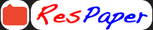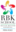Trending ▼   ResFinder# ICSE Class 8 Board Exam 2021 : Mathematics

4 pages, 5 questions, 0 questions with responses, 0 total responses,00Avrojit Dutta R. B. K. School (RBK), Mira Road
+Fave Message
 Home > avrojitdutta >Formatting page ...

ASSESSMENT 6 2020 21 Std: VIII Marks: 60 Date: 05/04/21 Subject: Mathematics Dur. : 1 Hours _________________________________________________________________________ Q.1. Factorise the following polynomials 1) a2 a(b+5) + 5b 2 2) 7+10 (X Y) 8 (X Y)2 3 Q.2.1) The area of a rectangle is 2X3 8X2 + 5X + 6 and one of its side is X 2. 3 Find the length of the adjacent side. 2) Solve the following using identities a) (208)2 2 b) 30.8 x 29.2 2 3) If a2 + b2 = 73 and ab = 24, Find a b Q.3. 1) Solve : a 2 a+4 = a 3 a+1 3 3 2) The denominator of a fraction is greater than its numerator by 7. If the numerator is increased by 4 and the denominator is decreased by 8, 4 8 the new fraction becomes . Find the original fraction. 3 3) Solve the inequation and represent its solution on the number line. 3X 8 7 , X W 1 3Formatting page ...Formatting page ...Formatting page ...